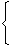# Aptitude :: Simplification

Exercise : Simplification - Formulas
1. 'BODMAS' Rule:

This rule depicts the correct sequence in which the operations are to be executed, so as to find out the value of given expression.

Here B - Bracket,
O - of,
D - Division,
M - Multiplication,
S - Subtraction

Thus, in simplifying an expression, first of all the brackets must be removed, strictly in the order (), {} and ||.

After removing the brackets, we must use the following operations strictly in the order:

(i) of (ii) Division (iii) Multiplication (iv) Addition (v) Subtraction.

2. Modulus of a Real Number:

Modulus of a real number a is defined as

 |a| =a, if a > 0 -a, if a < 0

Thus, |5| = 5 and |-5| = -(-5) = 5.

3. Virnaculum (or Bar):

When an expression contains Virnaculum, before applying the 'BODMAS' rule, we simplify the expression under the Virnaculum.